### Featured

October 22, 2020

In my last post I touched on the topic of continuously improving your geo-computing projects (also take a look at my chapter from the upcoming Software Underground book, 52 things you should know about geocomputing).

However, one aspect that I intentionally left out in was that of coding skills as I was planning to get back to it with a dedicated post, which you are reading just now.

#### 2018 vs 2020 comparison of flag percentage calculation

In the Jupyter notebook I compare the results of seismic inversion from two methods (with or without inversion-tailored noise attenuation) using a custom function to flag poor prediction of the target well log using median/median absolute deviation as a statistic for the error; the results are shown below.

One may just do this visual comparison, but I also included calculations to count the number and percentage of samples that have been flagged for each case. Below is a cell of code from the Jupyter notebook (let’s call it 2020 code) that does just that .

``zone_errors_a['flagged samples']=result_a.groupby('zone', sort=False).flag.sum().valueszone_errors_b['flagged samples']=result_b.groupby('zone', sort=False).flag.sum().valuesdef calc_proportion(dtf):    """    function to calculate proportion of flagged samples    """    x=dtf.flag    return round(100 * x.sum()/len(x), 1)zone_errors_a['proportion (%)']=result_a.groupby('zone',sort=False).apply(calc_proportion).valueszone_errors_b['proportion (%)']=result_b.groupby('zone',sort=False).apply(calc_proportion).values``

I am a lot happier with this code than with the original code (circa 2018), which is in the cell below.

``````zones_a=list(result_a['zone'].unique())
zones_b=list(result_b['zone'].unique())

zone_errors_a['flagged samples']=[result_a.loc[result_a.zone==z,'flag'].sum() for z in zones_a]
zone_errors_b['flagged samples']=[result_b.loc[result_b.zone==z,'flag'].sum() for z in zones_b]

zone_errors_a['proportion (%)']=[round(result_a.loc[result_a.zone==z,  'flag'].sum()/len(result_a.loc[result_a.zone==z,'flag'])*100,1) for z in zones_a]

zone_errors_b['proportion (%)']=[round(result_b.loc[result_b.zone==z,  'flag'].sum()/len(result_b.loc[result_b.zone==z,'flag'])*100,1) for z in zones_b]                                    ``````

The major differences in the older code are:

• I was using `unique` instead of Pandas’ `groupby`
• I was using list comprehensions to work through the DataFrame, instead of Pandas’ `apply` and a custom function to calculate the percentages on the entire DataFrame at once.

I find the 2020 code much more tidy and easier to read.

#### Enters Pandas for everyone

The above changes happened in but a few hours over two evenings, after having worked through chapters 9 and 10 of Pandas for Everyone by Daniel Chen, a very accessible read for all aspiring data scientists, which I highly recommend (also, watch Daniel’s fully-packed 2019 Pycon tutorial).

And before you ask: no, you do not get the Agile Scientific sticker with the book, I am sorry.

🙂

#### Comparison of 2016 vs 2020 code snippets from the 2016 SEG Machine Learning contest

A second example is of code used to calculate the first and second derivatives for all geophysical logs from the wells in the 2016 SEG Machine Learning contest.

The two cells of code below do exactly the same thing: loop through the wells and for each one in turn loop through the logs, calculate the derivatives, add them to a temporary Pandas DataFrame, then concatenate into a single output DataFrame. In this case, the only difference is the moving away from `unique` to `groupby`.

I use the `%%timeit` cell magic to compare the runtimes for the two cells.

###### 2016 code
``````%%timeit
# for training data
# calculate all 1st and 2nd derivative for all logs, for all wells
train_deriv_df = pd.DataFrame()             # final dataframe

for well in train_data['Well Name'].unique():        # for each well
new_df = pd.DataFrame() # make a new temporary dataframe

for log in ['GR', 'ILD_log10', 'DeltaPHI', 'PHIND' ,'PE']: # for each log
# calculate and write to temporary dataframe
new_df[str(log) + '_d1'] = np.array(np.gradient(train_feat_df[log][train_feat_df['Well Name'] == well]))

# append all rows of temporary dataframe to final dataframe
train_deriv_df = pd.concat([train_deriv_df, new_df])

86 ms ± 1.47 ms per loop (mean ± std. dev. of 7 runs, 10 loops each)``````
###### 2020 code
``````%%timeit
# for training data
# calculate all 1st and 2nd derivative for all logs, for all wells
train_deriv_df = pd.DataFrame() # final dataframe

for _, data in train_feat_df.groupby('Well Name'): # for each well
new_df = pd.DataFrame()                        # make a new temporary dataframe

for log in ['GR', 'ILD_log10', 'DeltaPHI', 'PHIND' ,'PE']: # for each log
# calculate and write to temporary dataframe

# append all rows of temporary dataframe to final dataframe
train_deriv_df = pd.concat([train_deriv_df, new_df])

52.3 ms ± 353 µs per loop (mean ± std. dev. of 7 runs, 10 loops each)``````

We go down to 52.3 ms from 86 ms, which is a modest improvement, but certainly the code is more compact and a whole lot lighter to read (i.e. more pythonic, or pandaish if you prefer): I am happy!

As an aside, if you want to know more about timing code execution, see section 1.07 from Jake VanderPlas’ outstanding Python Data Science Handbook, which I also cannot recommend enough (and do yourself a favor: watch his series Reproducible Data Analysis in Jupyter).

By the way, below I show the notebook code comparison generated using the nbdiff-web option from the awesome `nbdime` library, a recent discovery.

# Busting bad colormaps with Python and Panel

I have not done much work with, or written here on the blog about colormaps and perception in quite some time.

Last spring, however, I decided to build a web-based app to show the effects of using a bad colormaps. This stemmed from two needs: first, to further my understanding of Panel, after working through the awesome tutorial by James Bednar, Panel: Dashboards (at PyData Austin 2019); and second, to enable people to explore interactively the effects of bad colormaps on their perception, and consequently the ability to on interpret faults on a 3D seismic horizon.

I introduced the app at the Transform 2020 virtual subsurface conference, organized by Software Underground last June. Please watch the recording of my lightning talk as it explains in detail the machinery behind it.

I am writing this post in part to discuss some changes to the app. Here’s how it looks right now: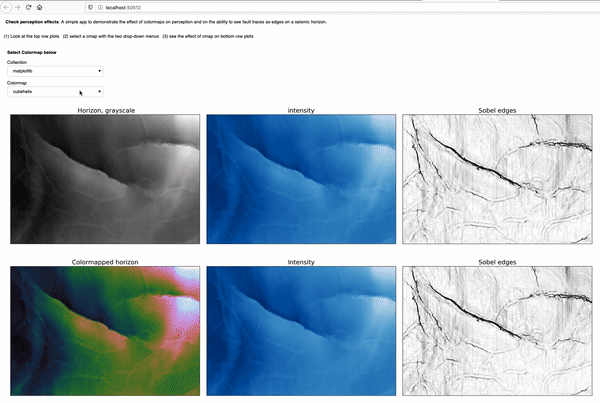The most notable change is the switch from one drop-down selector to two-drop-down selectors, in order to support both the Matplotlib collection and the Colorcet collection of colormaps. Additionally, the app has since been featured in the resource list on the Awesome Panel site, an achievement I am really proud of.You can try the app yourself by either running the notebook interactively with Binder, by clicking on the button below:```https://mybinder.org/v2/gh/mycarta/Colormap-distorsions-Panel-app/master?urlpath=%2Fpanel%2FDemonstrate_colormap_distortions_interactive_Panel
```

Let’s look at a couple of examples of insights I gained from using the app. For those that jumped straight to this example, the top row shows:

• the horizon, plotted using the benchmark grayscale colormap, on the left
• the horizon intensity, derived using `skimage.color.rgb2gray`, in the middle
• the Sobel edges detected on the intensity, on the right

and the bottom row,  shows:

• the horizon, plotted using the Matplotlib `gist_rainbow` colormap, on the left
• the intensity of the colormapped, in the middle. This is possible thanks to a function that makes a figure (but does not display it), plots the horizon with the specified colormap, then saves plot in the canvas to an RGB numpy array
• the Sobel edges detected on the colormapped intensity, on the rightI think the effects of this colormaps are already apparent when comparing the bottom left plot to the top left plot. However, simulating perception can be quite revealing for those that have not considered these effects before. The intensity in the bottom middle plot is very washed out in the areas corresponding to green color in the bottom left, and as a result, many of the faults are not visible any more, or only with much difficulty, which is demonstrated by the Sobel edges in the bottom right.

And if you are not quite convinced yet, I have created these hill-shaded maps, using Matt Hall”s delightful function from this notebook (and check his blog post):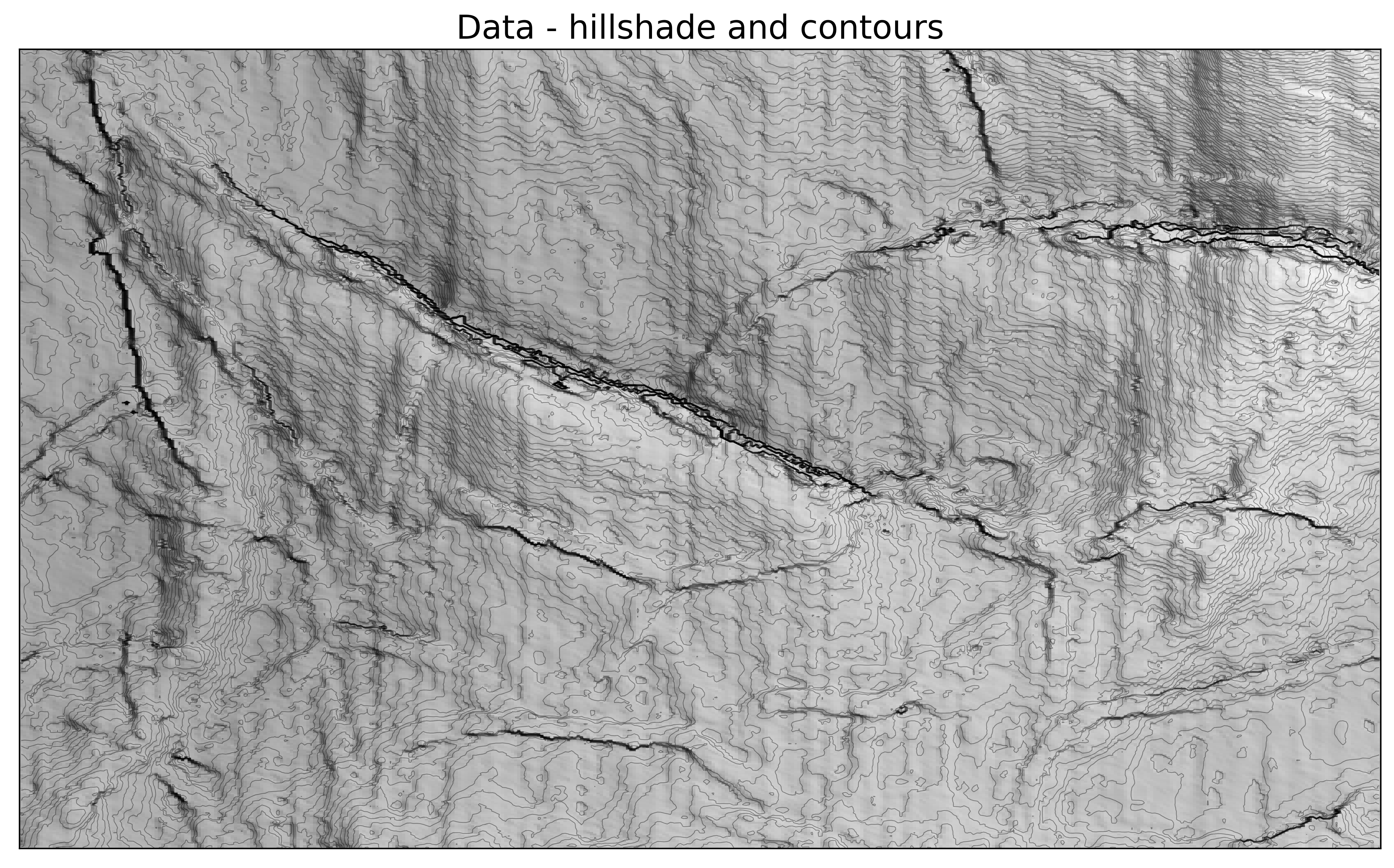Below is another example, using the Colocrcet `cet_rainbow` which is is one of Peter Kovesi’s perceptually uniform colormaps.  I use many of Peter’s colormaps, but never used this one, because I use my own perceptual rainbow, which does not have  a fully saturated yellow, or a fully saturated red. I think the app demonstrate, that even though they are more subtle , this rainbow still is introducing some artifacts. The yellow colour creates narrow flat bands, visible in the intensity and Sobel plots, and indicated by yellow arrows; the red colour is also bad as usual, causing an artificial decrease in intensity(magenta arrows).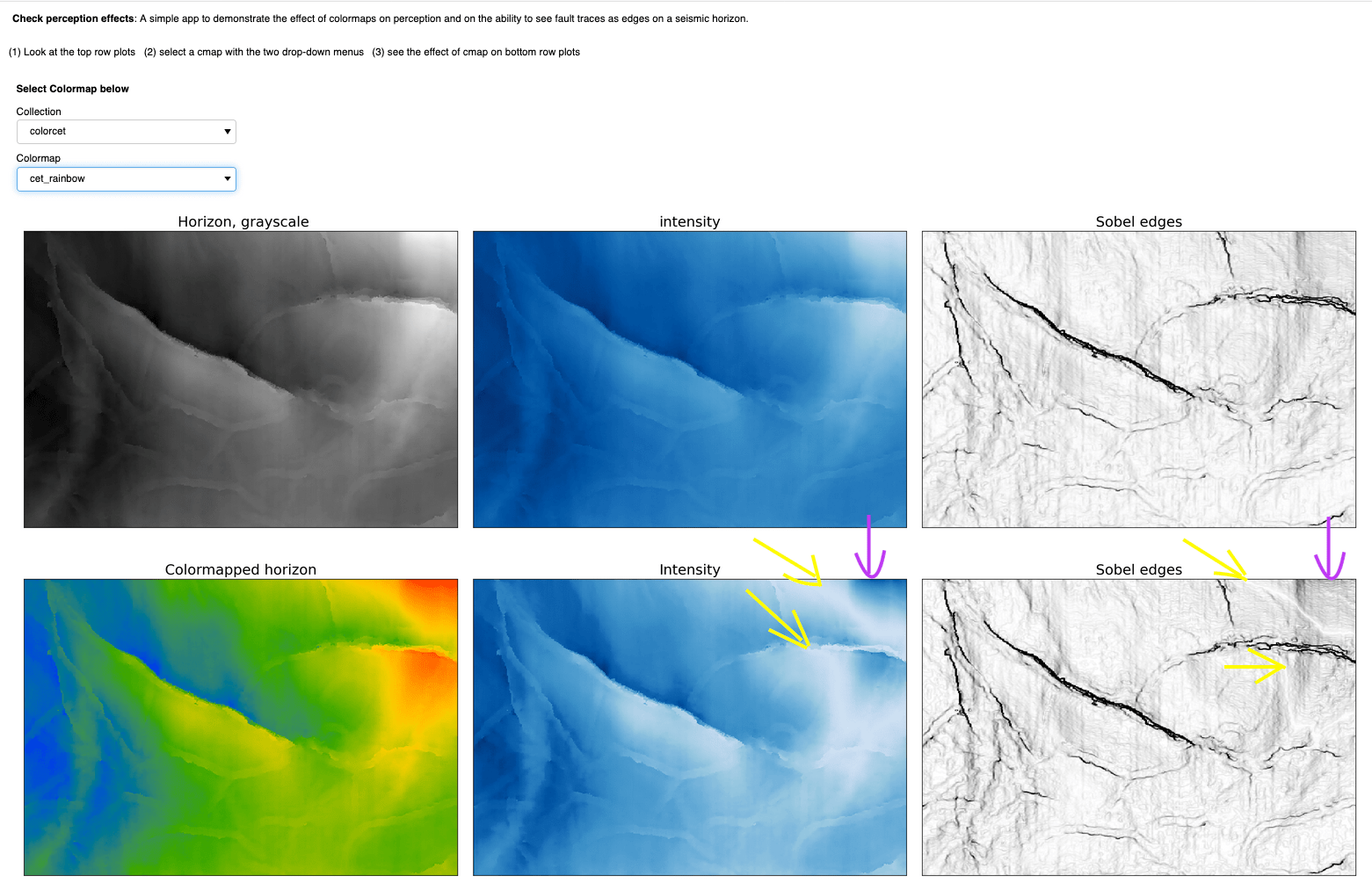## Be a geoscience and data science detective

### Featured

September 16, 2020

#### Introduction

These days everyone talks about data science. But here’s a question: if you are a geoscientist, and like me you have some interest in data science (that is, doing more quantitative and statistical analyses with data), why choose between the two? Do both… always! Your domain knowledge is an indispensable condition, and so is an attitude of active curiosity (for me, an even more important condition). So, my advice for aspiring geoscientists and data scientists is: “yes, do some course work, read some articles, maybe a book, get the basics of Python or R, if you do not know a programming language” but then jump right ahead into doing! But – please! – skip the Titanic, Iris, Cars datasets, or any other data from your MOOC or that many have already looked at! Get some data that is interesting to you as geoscientist, or put it together yourself.

#### First example: evaluate the quality of seismic inversion from a published article

In this first example, the one presented at Transform 2020, I wanted to evaluate the quality of seismic inversion from a published article in the November 2009 CSEG Recorder Inversion Driven Processing. In the article, the evaluation was done by the authors at a blind well location, but only qualitatively, as illustrated in Figure 5, shown below for reference. In the top panel (a) the evaluation is for the inversion without additional processing (SPNA, Signal Protected Noise Attenuation); in the bottom panel (b) the evaluation is for the inversion with SPNA. On the right side of each panel the inverted seismic trace is plotted against the upscaled impedance well log (calculated by multiplying the well density log and the well velocity log from compressional sonic); on the right, the upscaled impedance log is inserted in a seismic impedance section as a colored trace (at the location of the well) using the same color scale and range used for the impedance section.Figure 5 caption: Acoustic impedance results at the blind well for data without (a) and with (b) SPNA. The figure shows a 200 ms window of inverted seismic data with well B, the blind well, in the middle on the left, along with acoustic impedance curves for the well (red) and inverted seismic (blue) on the right. The data with SPNA shows a better fit to the well, particularly over the low frequencies.

What the authors reported in the figure caption is the extent to which the evaluation was discussed in the paper; unfortunately it is not backed up in any quantitative way, for example comparing a score, such as R^2, for the two methods. Please notice that I am not picking on this paper in particular, which in fact I rather quite liked, but I am critical of the lack of supporting statistics, and wanted to supplement the paper with my own.

In order to do that, I hand-digitized from the figure above the logs and inversion traces , then interpolated to regularly-sampled time intervals (by the way: if you are interested in a free tool to  digitize plots, check  use WebPlotDigitizer).

My plan was to split my evaluation in an upper and lower zone, but rather than using the seismically-picked horizon, I decided to add a fake top at 1.715 seconds, where I see a sharp increase in impedance in Figure 5. This was an arbitrary choice on my part of a more geological horizon, separating the yellow-red band from the green blue band in the impedance sections. The figure below shows all the data in a Matplotlib figure:The first thing I did then, was to look at the Root Mean Square Error in the upper and lower zone obtained using the fake top. They are summarized in the table below: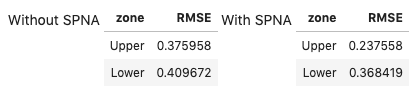Based on the RMSE , it looks like case b, the inversion with Signal Protected Noise Attenuated applied on the data, is a better result for the `Upper` zone, but not for the `Lower` one. This result is in agreement with my visual comparison of the two methods.

But lets’ dig a bit deeper. After looking at RMSE, I used the updated version of the `error_flag` function, which I first wrote at the 2018 Geophysics Sprint, listed below:

```def error_flag(pred, actual, stat, dev = 1.0, method = 1):
"""Calculate the difference between a predicted and an actual curve
and return a curve flagging large differences based on a user-defined distance
(in deviation units) from either the mean difference or the median difference

Matteo Niccoli, October 2018. Updated in May 2020.

Parameters:
predicted : array
predicted log array
actual : array
original log array
stat : {‘mean’, ‘median’}
The statistics to use. The following options are available:
- mean: uses numpy.mean for the statistic,
and np.std for dev calculation
- median: uses numpy.median for the statistic,
and scipy.stats.median_absolute_deviation (MAD) for dev calculation
dev : float, optional
the standard deviations to use. The default is 1.0
method : int {1, 2, 3}, optional
The error method to use. The following options are available
(default is 1):
1: difference between curves larger than mean difference plus dev
2: curve slopes have opposite sign (after a 3-sample window smoothing)
3: curve slopes of opposite sign OR difference larger than mean plus dev
Returns:
flag : array
The error flag array
"""

flag = np.zeros(len(pred))
err = np.abs(pred-actual)

if stat == 'mean':
err_stat = np.mean(err)
err_dev = np.std(err)
elif stat == 'median':
err_stat = np.median(err)
err_dev = sp.stats.median_absolute_deviation(err)

pred_sm = pd.Series(np.convolve(pred, np.ones(3), 'same'))
actual_sm = pd.Series(np.convolve(actual, np.ones(3), 'same'))
ss = np.sign(pred_sm.diff().fillna(pred_sm))
ls = np.sign(actual_sm.diff().fillna(actual_sm))

if method == 1:
flag[np.where(err>(err_stat + (dev*err_dev)))] = 1
elif method == 2:
flag[np.where((ss + ls)==0 )]= 1
elif method == 3:
flag[np.where(np.logical_or(err>(err_stat + (dev*err_dev)), (ss+ls)==0 ))]= 1
return flag```

I believe this new version is greatly improved because:

• Users now can choose between mean/standard deviation and median/median absolute deviation as a statistic for the error. The latter is more robust in the presence of outliers
• I added a convolutional smoother prior to the slope calculation, so as to make it less sensitive to noisy samples
• I expanded and improved the doctstring

The figure below uses the flag returned by the function to highlight areas of poorer inversion results, which I assigned based on passed to the function very restrictive parameters:

• using median and a median absolute deviation of 0.5 to trigger flag
• combining the above with checking for the slope signI also wrote short routines to count the number and percentage of samples that have been flagged, for each result, which are summarized in the table below: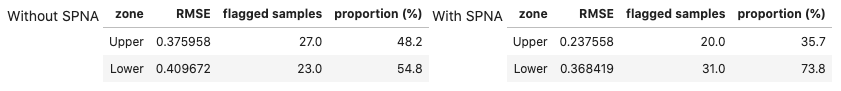The error flag method is in agreement with the RMS result: case b, the inversion on Signal Protected Noise Attenuated data is a better result for the `Upper` zone, but for the `Lower` zone the inversion without SPNA is the better one. Very cool!

But I was not satisfied yet. I was inspired to probe even deeper after a number of conversations with my friend Thomas Speidel, and reading the chapter on Estimation from Computational and Inferential Thinking (UC Berkeley). Specifically, I was left with the question in mind: “Can we be confident about those two is better in the same way?

This question can be answered with a bootstrapped Confidence Interval for the proportions of flagged samples, which I do below using the code in the book, with some modifications and some other tools from the datascience library. The results are shown below. The two plots, one for the `Upper` and one for the `Lower` zone, show the distribution of bootstrap flagged proportions for the two inversion results, with SPNA in yellow, and without SPNA in blue, respectively, and the Confidence Intervals in cyan and brown, respectively (the CI upper and lower bounds are also added to the table, for convenience).

By comparing the amount (or paucity) of overlap between the distributions (and between the confidence intervals) in the two plots, I believe I can be more confident in the conclusion drawn for the Lower zone, which is that the inversion on data without SPNA is better (less proportion of flagged errors), as there is far less overlap.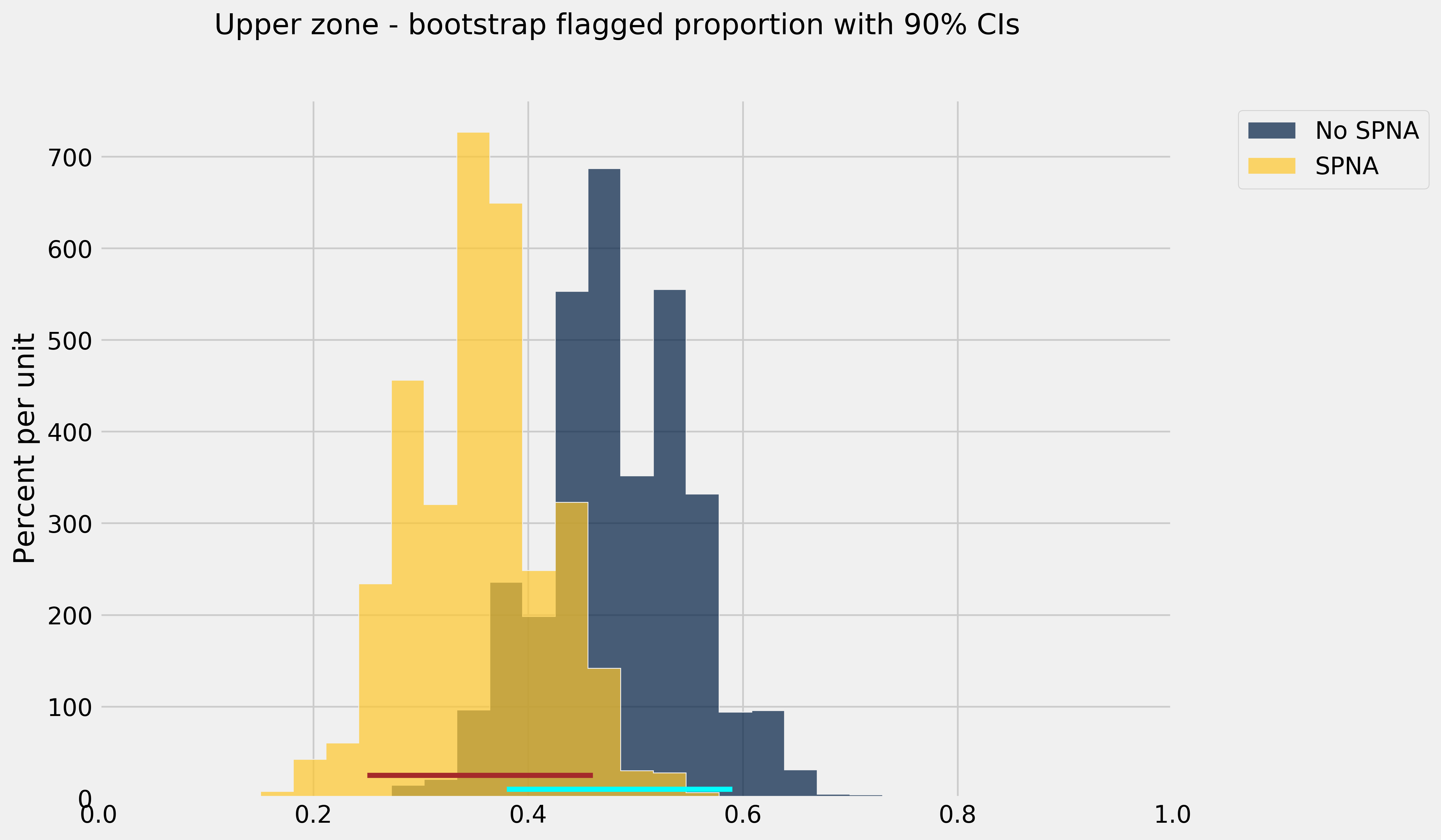I am very pleased with these results. Of course, there are some caveats to keep in mind, mainly that:
• I may have introduced small, but perhaps significant errors with hand digitizing
• I chose a statistical measure (the median and median absolute deviation) over a number of possible ones
• I chose an arbitrary geologic reference horizon without a better understanding of the reservoir and the data, just the knowledge from the figure in the paper

However, I am satisfied with this work, because it points to a methodology that I can use in the future. And I am happy to share it! The Jupyter notebook is available on GitHub.

#### Second example: evaluate regression results from a published article

My second, more recent example, is shorter but no less interesting, in my opinion. It is a case study correlating inversion data to petrophysical estimates of porosity-height in the Doig and Montney Formations in Western Canada (from the paper Tight gas geophysics: AVO inversion for reservoir characterization, Close et al. CSEG Recorder, May 2010, an article which I enjoyed very much reading).

The authors indicated that Vp/Vs and/or Poisson’s ratio maps from seismic inversion are good indicators of porosity in the Lower Doig and Upper Montney reservoirs in the wells used in their study, so it was reasonable to try to predict Phi-H from Vp/Vs via regression. The figure below, from the article, shows one such Vp/Vs ratio map and the Vp/Vs vs. Phi-H cross-plot for 8 wells.Figure 7 caption: Figure 7. a) Map of median Vp/Vs ratio value and porosity-height from 8 wells through the Lower Doig and Upper Montney. The red arrows highlight wells with very small porosity-height values and correspond in general to areas of higher Vp/Vs ratio. The blue arrow highlights a well at the edge of the seismic data where the inversion is adversely affected by decreased fold. The yellow line is the approximate location of a horizontal well where micro-seismic data were recorded during stimulation. b) Cross-plot of porosity-height values as shown in (a) against Vp/Vs extracted from the map. The correlation co-efficient of all data points (blue line) of 0.7 is improved to 0.9 (red line) by removing the data point marked by the blue arrow which again corresponds to the well near the edge of the survey (a).

They also show in the figure that by removing one of the data points, corresponding to a well near the edge of the survey (where the seismic inversion result is presumably not as reliable, due to lower offset and azimuth coverage), the correlation co-efficient is improved from 0.7 to 0.9 (red line).

So, the first thing I set out to do was to reproduce the crossplot.  I again hand-digitized the porosity-height and Vp/Vs pairs in the cross-plot using again WebPlotDigitizer. However, switched around the axes, which seems more natural to me since the objectives of regression efforts would be to predict as the dependent variable Phi-h, at the location of yet to drill wells, given Vp/Vs from seismic inversion. I also labelled the wells using their row index, after having loaded them in a Pandas DataFrame. And I used Ordinary Least Square Regression from the `statsmodels` library twice: once with all data points, the second time after removal of the well labelled as 5 in my plot above.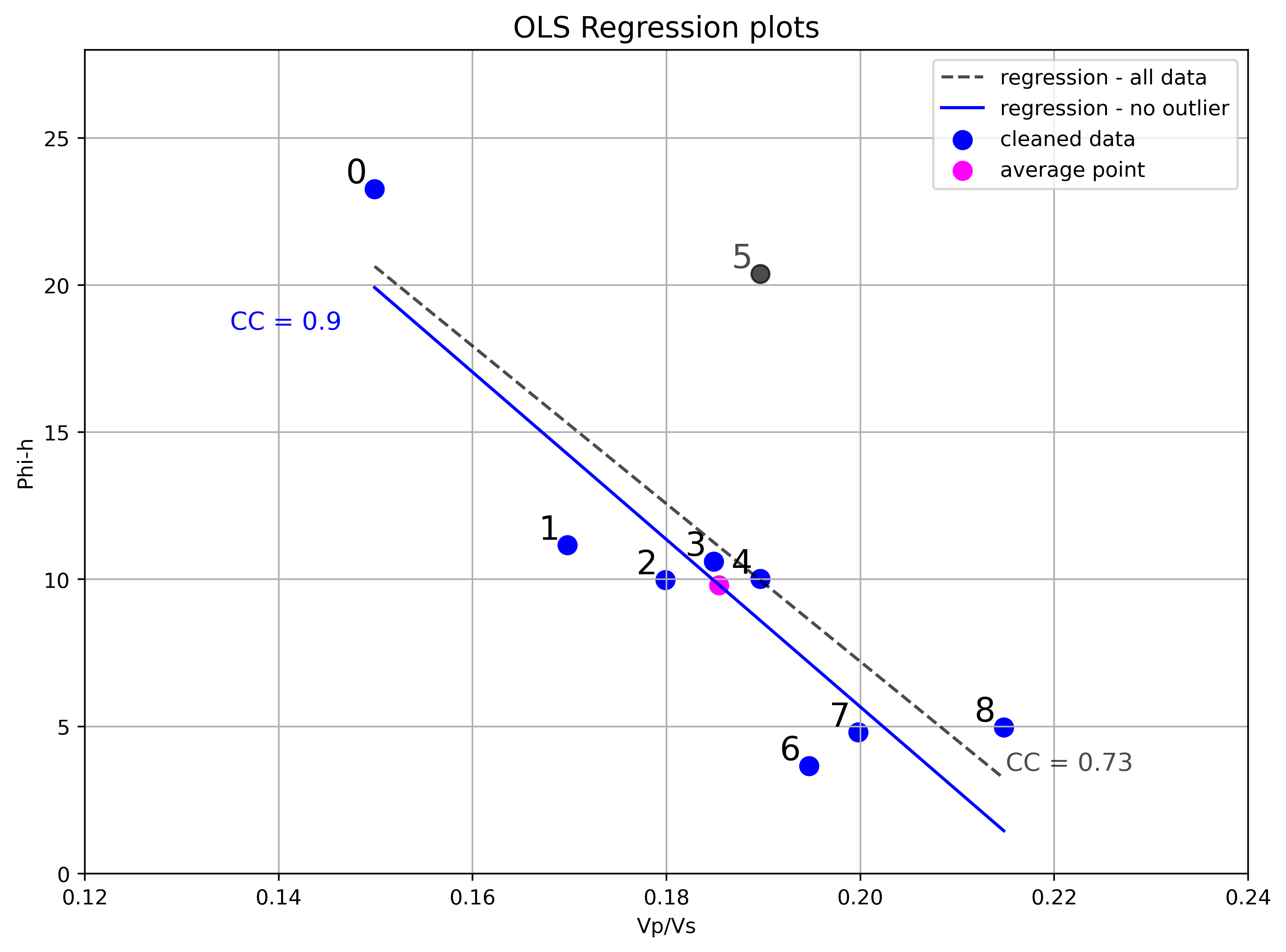So, I am able to reproduced the analysis from the figure. I think removing an outlier with insight from domain knowledge (the observation that poorer inversion result at this location is reasonable for the deviation from trend) is a legitimate choice. However, I would like to dig a bit deeper, to back up the decision with other analyses and tests, and to show how one might do it with their own data.

The first thing to look at is an Influence plot, which is a plot of the residuals, scaled by their standard deviation, against the leverage, for each observation. Influence plots are useful to distinguish between high leverage observations from outliers and are one of `statsmodel` ‘s standard Regression plots, so we get the next figure almost for free, with minor modifications to the default example). Here it is below, together with the OLS regression result, with all data points.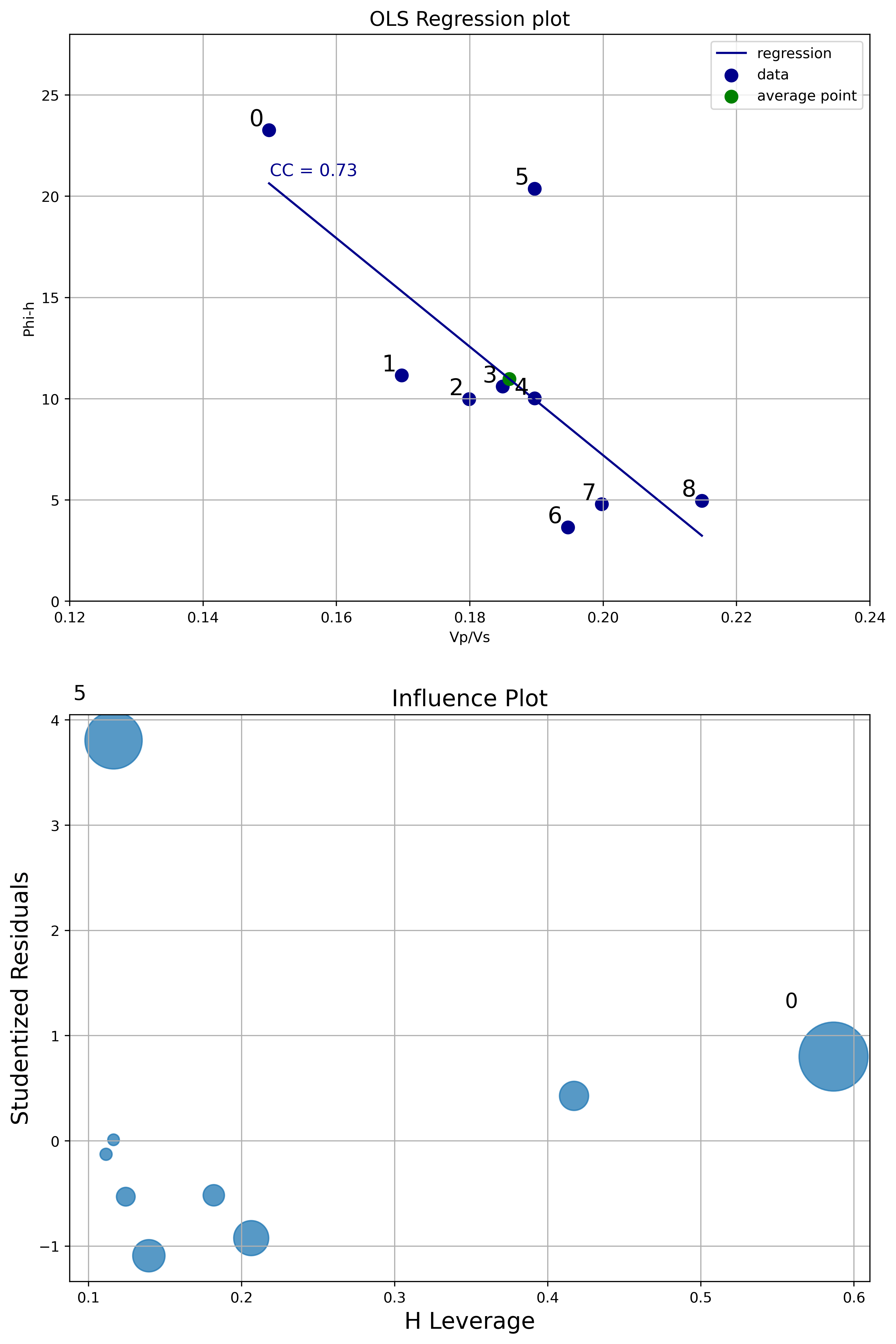From the Influence plot it is very obvious that the point labelled as zero has high leverage (but not high normalized residual). This is not a concern because points with high leverage are important but do not alter much the regression model. On the other hand, the point labelled as 5 has very high normalized residual. This point is an outlier and it will influence the regression line, reducing the R^2 and correlation coefficient. This analysis is a robust way to legitimize removing that data point.

Next I run some inferential tests. As I’ve written in an earlier notebook on Data loading, visualization, significance testing, I find the critical r very useful in the context of bivariate analysis. The critical r is the value of the correlation coefficient at which you can rule out chance as an explanation for the relationship between variables observed in the sample, and I look at it in combination with the confidence interval of the correlation coefficient.

The two plots below display, in dark blue and light blue respectively, the upper and lower confidence interval bounds, as the correlation coefficient r varies between 0 and 1 (x axis). These two bounds will change with different number of wells (they will get closer with more wells, and farther apart with less wells). The lower bound intersects the x axis (y=0) axis at a value equal to the critical r (white dot). The green dots highlight the actual confidence interval for a specific correlation coefficient chosen, in this case 0.73 with 9 wells, and 0.9 with 8 wells.

By the way: these plots are screen captures from the interactive tool I built taking advantage of the Jupyter interactive functionality (`ipywidgets`). You can try the tool by running the Jupyter notebook.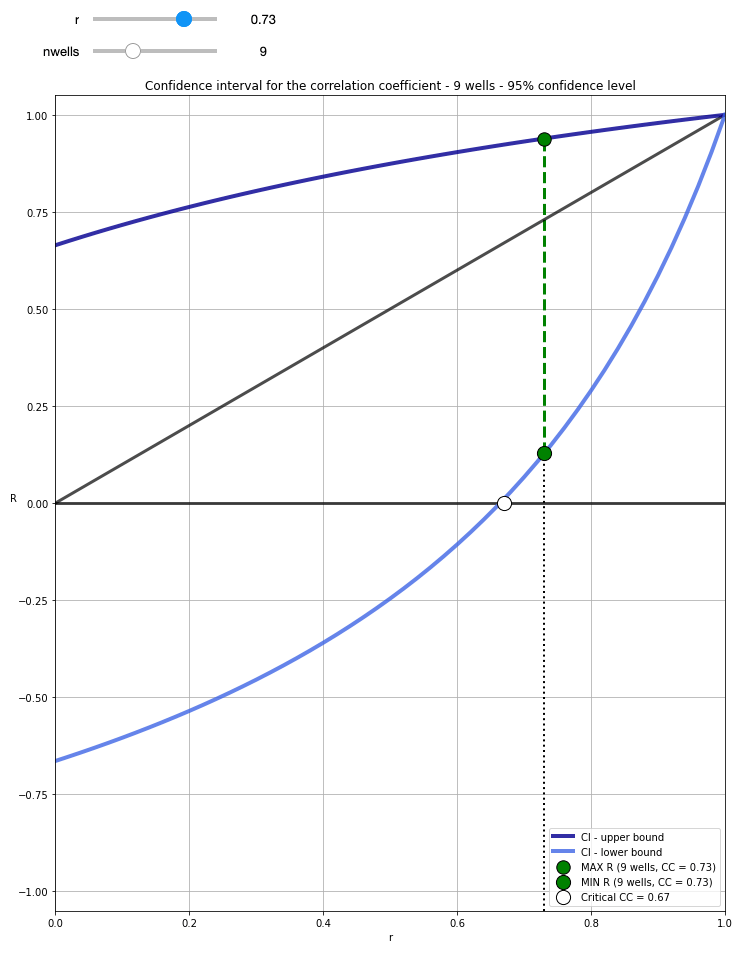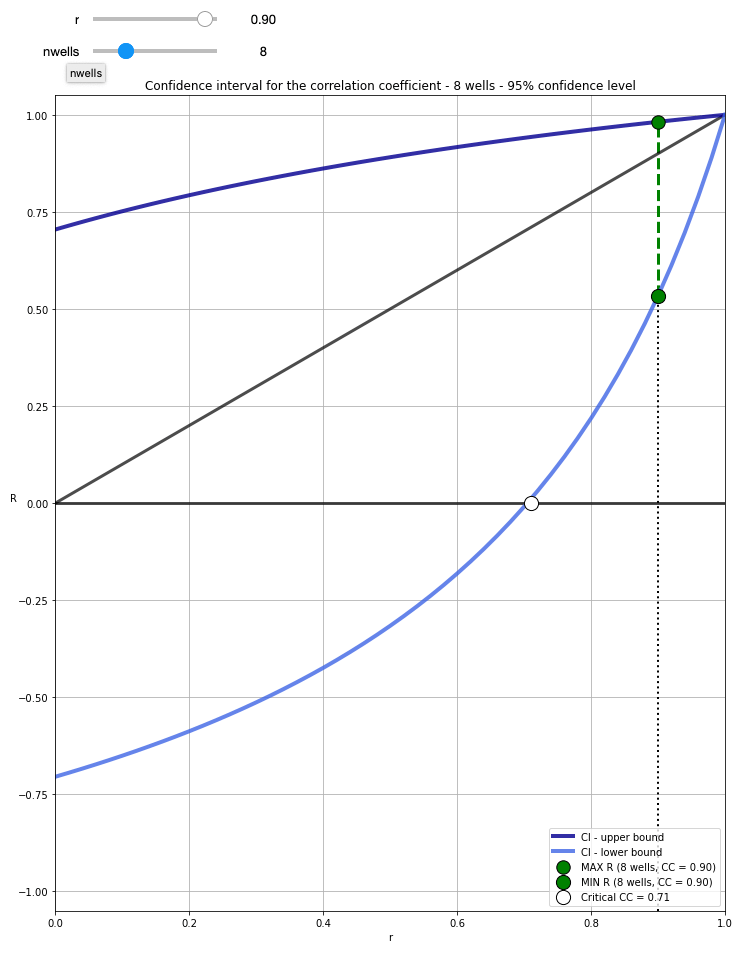With 9 wells, and cc=0.73, the resulting critical r = 0.67 tells us that for a 95% confidence level (0.05 alpha) we need at least a correlation coefficient of 0.67 in the sample (the 9 wells drilled) to be able to confidently say that there is correlation in the population (e.g. any well, future wells). However, the confidence interval is quite broad, ranging between 0.13 and 0.94 (you can get these numbers by running `confInt(0.73, 9)` in a cell.

With 8 wells (having removed the outlier), CC=0.9, the critical r is now 0.71, meaning that the requirement for rejecting the the null hypothesis (there is no association between Vp/Vs and Phi-H) is now a bit higher. However, a CC increased to 0.9, and only one less well, also results in a confidence interval ranging from 0.53 to 0.98, hence our confidence is greatly increased.

This second analysis also corroborates the choice of removing the outlier data point. One thing worth mentioning before moving on to the next test is that these confidence interval bounds are the expected population ones, based on the sample correlation coefficient and the number of observations; the data itself was not used. Of course, I could also have calculated, and shown you, the OLS regression confidence intervals, as I have done in this notebook (with a different dataset).

My final test involved using the distance correlation (`dcor.distance_correlation`) and p-value (`dcor.independence.distance_covariance_test`) from the `dcor` library. I have written before in a blog post how much I like the distance correlation, because it does not assume a linear relationship between variables, and because a distance correlation of zero does mean that there is no dependence between those two variables (contrary to Pearson and Spearman). In this case the relationship seems to be linear, but it is still a valuable test to compare DC and p-value before and after removing the outlier. Below is a summary of the test:
```All data points:
D.C. =  0.745
p-value =  0.03939
```
```Data without outlier:
D.C. =  0.917
p-value =  0.0012
```

The distance correlation values are very similar to the correlation coefficients from OLS, again going up once removed the outlier. But, more interestingly, with all data points, a p-value of 0.04079 is very close to the alpha of 0.05, whereas once we removed the outlier, the p-value goes down by a factor of 20, to 0.0018. This again backs up the decision to remove the outlier data point.

# Variable selection in Python, part I

#### Introduction and recap

In my previous two posts of this (now official, but) informal Data Science series I worked through some strategies for doing visual data exploration in Python, assisted by domain knowledge and inferential tests (rank correlation, confidence, spuriousness), and then extended the discussion to more robust approaches involving distance correlation and variable clustering.

For those that have not read those posts, I am using a dataset comprising 21 wells producing oil from a marine barrier sand reservoir; the data was first published by Lee Hunt in 2013 in a CSEG Recorder paper titled Many correlation coefficients, null hypotheses, and high value.

Oil production, the dependent variable, is measured in tens of barrels of oil per day (it’s a rate, actually). The independent variables are: Gross Pay, in meters; Phi-h, porosity multiplied by thickness, with a 3% porosity cut-off; Position within the reservoir (a ranked variable, with 1.0 representing the uppermost geological facies, 2.0 the middle one, 3.0 the lowest one); Pressure draw-down in MPa. Three additional ‘special’ variables are: Random 1 and Random 2, which are range bound and random, and were included in the paper, and Gross Pay Transform, which I created specifically for this exercise to be highly correlated to Gross pay, by passing Gross pay to a logarithmic function, and then adding a bit of normally distributed random noise.

#### Next step: variable selection (Jupyter Notebooks here)

The idea of variable selection is to try to understand which independent variables are more and which are less important in predicting the dependent variable (Production in this case), and also which ones may be highly correlated to one another (in other words, they carrying the same information); in both cases, assisted by domain knowledge, we drop some of the variables, resulting (ideally) in an improved prediction by a model that is simpler and can generalize better.

I really love the systematic way in which Thomas, working on the same dataset but using R, looked at several methods for variable selection and then summarized all the results in a table. The insight from this (quite) exhaustive analysis helped him chose a subset of variables to use in the final regression. I really, REALLY recommend reading his interactive R notebook.

As for me, one of the goals I had in mind at the end of our 2018 collaboration on this project was to be able to do something similar in Python, and I am delighted to say I think I was able to achieve that goal.

In this post I will look at:

• Distance correlation, again
• Multicollinearity, using Variance Inflation Factor (VIF)
• Sequential feature selection, using both a backward and forward approach
• Random Forest Regressor variable importance, using a drop-column approach
• Multicollinearity, using variable dependence

In the next (1 or 2) post(s) I will look at:

• Permutation importance using an Extra Tree Regressor
• Mutual information
• The relative magnitude of the transformed variables in ACE (Alternating Conditional Expectation)
• SHAP values (Shapley additive explanations)
• The sign of the weights of a neural network (excitory (positive weights) vs. inhibitory (negative weighs))

I think this is a good mix as it combines methods and then summarize the results from all methods.

##### Distance correlation

in Figure 1, below, I plot again the correlation matrix of bivariate scatterplots, rearranged according to the clustering results from last post, and with the distance correlation annotated and coloured by its bootstrapping p-value.

Phi-h, Gross Pay, and Gross pay transform are highly correlation to Production, with statistical significance at the 10%level given by the p-value. However, there is a good chance also also of multicollinearity at play, almost certainly between Gross Pay and Gross Pay Transform, with a DC of 0.97; we know why, in this case, imposed it in this case, but we might have not known.Figure 1. Seaborn pairgrid matrix with distance correlation colored by p-value (gray if > 0.10, blue if p <= 0.10), and plots rearranged by clustering results

##### Variance Inflation Factor (VIF)

Variance inflation factor (VIF) is a technique to estimate the severity of multicollinearity among independent variables within the context of a regression. It is calculated as the ratio of all the variances in a model with multiple terms, divided by the variance of a model with one term alone.

First, we set-up a regression, using the Patsy library to generate design matrices for target and predictors:

```outcome, predictors = dmatrices("Production ~ Gross_pay +Phi_h +Position
+Pressure +Random1 +Random2 +Gross_pay_transform",
data, return_type='dataframe')
```

for which then VIF factors can be calculated with:

```vif["VIF Factor"] = [variance_inflation_factor(predictors.values, i)
for i in range(predictors.shape)]```

The values are summarized in Table I below; variables that have variance inflation factor that is high (ignoring the intercept) and similar in value have a high chance of being collinear because they explain the same variance in the dataset.Table I. Regression VIF factors

For this model, the result suggests either Gross Pay or Gross Pay Transform should be dropped, otherwise the risk is of building a model with high multicollinearity (that is, predictions would be very susceptible to small noise fluctuations).

But which one should we drop? It occurred to me that one possibility would be to drop one in turn and recalculate the VIF factors.Table II. VIF after dropping Gross Pay Transform

As seen in Table II, after removing Gross Pay Transform  all VIF factors are below the cut-off value of 5 (rule-of-thumb suggested in this article, and reference therein). I  would make the additional observation. that because the factors for Phi-h and Gross Pay are now close, even though below the cutoff,  there may be some (smaller amount of) collinearity between the two variables, which is consistent to be expected since both variables contain some information on height (one of pay, one of porosity).

We see something similar when removing Gross Pay; in fact, the Factors for Gross Pay Transform and Phi-h in Table III are also close, yes, but smaller. I’d conclude that VIF is veru sueful in highlighting multicollinearity, but it does not necessarily answer the question of which collinear feature shoud be dropped.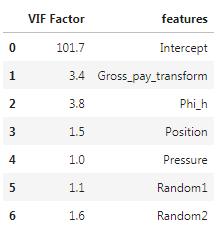Table III. VIF after dropping Gross Pay

##### Sequential feature selection

Sequential feature selection (similarly to Scikit-learn’s Recursive Feature Elimination) is used  “to automatically select a subset of features that is most relevant to the problem. The goal of feature selection is two-fold: we want to improve the computational efficiency and reduce the generalization error of the model by removing irrelevant features or noise”.

I tested both Sequential Forward Selection (SFS) and Sequential Backward Selection (SBS) from Sebastan Raschka‘s  `mlxtend` library to search for that optimal subset of features (for a full overview of the method, and a great set of detailed examples, please see the excellent documentation by Sebastian). You can download and run the full notebook fro the GitHub repo here).

The only difference between SFFS and SBFS is that the former starts with at 1 feature and adds them one by one, whereas the latter starts with all features (or a user defined pre-selected number) and removes them one by one. In both cases I used the selector as part of a pipeline including Scikit-learn’s linear regression and cross-validation with Leave One Out (i.e., dropping one well at a time); for example, the pipeline for SFS is:

```features = data.loc[:, ['Position', 'Gross pay', 'Phi-h', 'Pressure',
'Random 1', 'Random 2', 'Gross pay transform']].values
y = data.loc[:, ['Production']].values

LR = LinearRegression()
loo = LeaveOneOut()

sfs = SFS(estimator=LR,
k_features=7,
forward=True,
floating=True,
scoring='neg_mean_squared_error',
cv = loo,
n_jobs = -1)
sfs = sfs.fit(X, y)
```

and the feature selection results are plotted in Figure 2, generated with a modified version of Sebastian’s `mlxtend` utility function:

```plot_sequential_feature_selection(sfs.get_metric_dict(), kind='std_err')
plt.gca().invert_yaxis()
```

Please notice that having flipped the y axis (my personal preference), performance for SFFS (as given by negative mean square error) improves towards the bottom.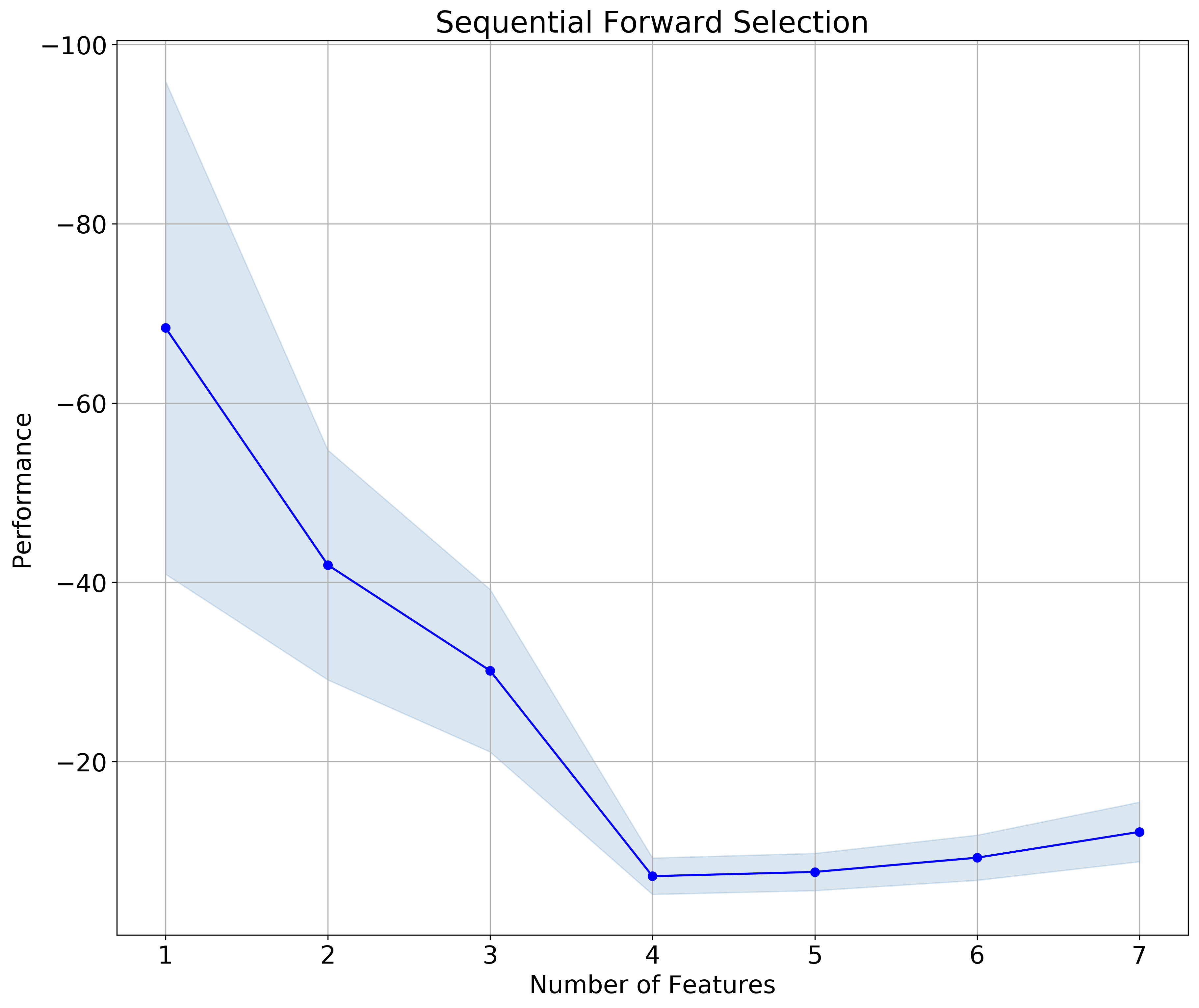Figure 2. Sequential Forward Selection

The results for SFBS is plotted in Figure 3. Notice that in this case I flipped both the y axis  and the x axis; the latter makes the sequential selection go from left to right, which I find a bit more intuitive, given we read from left to right.Figure 3. Sequential Backward Selection

In both cases the subset is made up of 4 feature, and – to my delight !! –  the selected features are the same (check the notebook to see how I extract the information):

```>>>  ['Position', 'Gross pay', 'Phi-h', 'Pressure']
```
##### Drop-column feature importance

You can download the notebook for both drop-column importance and dependence from here.

I have to say I’ve never been comfortable with using Feature Importance plots you get from Random Forest. In part because, on occasion, I noticed a disconnect with what domain knowledge-informed intuition would suggest; in part, I confess, because I thought (and I was right) I had an incomplete understanding of what goes on in the background. Until I read the article How to not use random forest. The example with toy dataset in there is not the most exciting, but it demonstrate clearly how using Feature Importance with preset parameters places a random variable at the top. If you wonder how can that be, I recommend reading the article.

Or read on, there’s more coming: curious, I did some more searching, and found this article, Selecting good features – Part III: random forests. There’s a nicer example in there, using the Boston Housing dataset, and to me a clearer explanation of why one should not use the default Scikit-learn Mean Decrease Impurity metric (strong, but correlated features can end up with low scores).

Finally, I found Beware Default Random Forest Importances, where the authors (thank you!!!) not only walk readers through a full set of experiments, run in both Python and R, but provide a great library (called `rfpimp`), to do your own work in Python.

I really like their drop-column importance, which is implemented to answers the question of how important a feature is to the overall model performance … and does it  … even more directly than the permutation importance.

That is achieved with a brute force drop-column apprach involving:

• training the model with all features to get a baseline performance score
• dropping a column
• retraining  the model and recomputing the performance score.

The importance value of a feature is then the difference between the baseline and the score from the model without that feature.

I also REALLY like that unimportant features do not have just very low importance; some do, but some have negative importance, exposing that removing them improves model performance. This is the case, with our small dataset of the Random 1 and Random 2 variables, as shown in Figure 4. It is also the case of Pressure. Of the remaining variables, Gross Pay Transform has very low importance (please notice the range is 0-0.15 for this plot, a conscious choice by the authors), Gross pay and Phi-h look somewhat important, and Position in the reservoir is the most important feature. This is excellent insight; please compare to the importances with Scikit-learn’s defautl metric, in Figure 5.Figure 4. rfpimp Drop-column importance. Notice the 0-0.15 range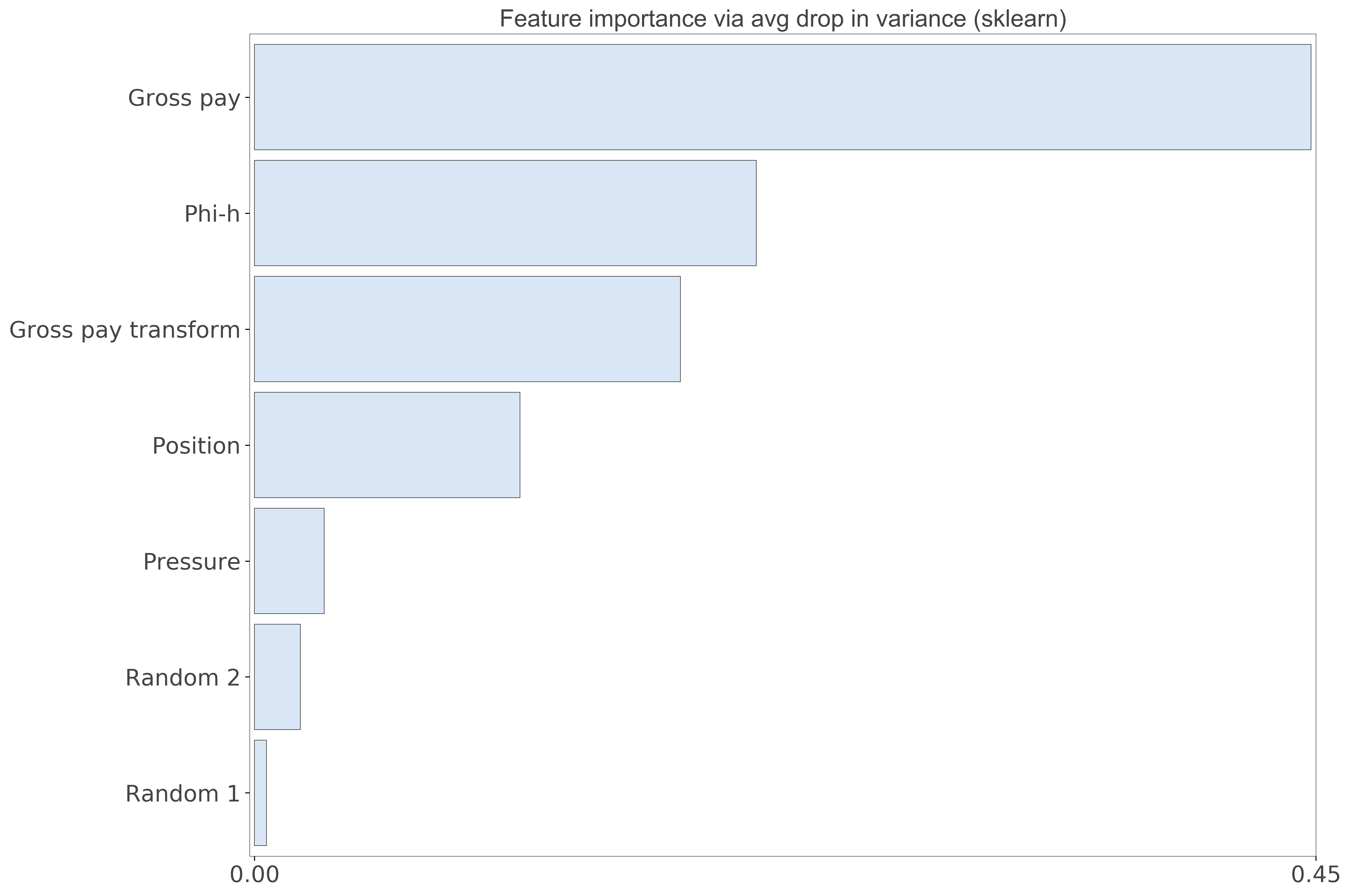Figure 5. Scikit-learn Feature importance. Notice the 0-0.45 range

##### Dependence

This last analysis is similar to Thomas’ Redundancy Analysis in that we look for those variables that can be predicted using the other variables.  Using the `feature_dependence_matrix` function from the `rfpimp` library we get:

```>>> Dependence:
Gross pay               0.939
Gross pay transform     0.815
Phi-h                   0.503
Random 2               0.0789
Position               0.0745
Pressure               -0.396
Random 1               -0.836

```

By removing Gross Pay Transform, and repeating the analysis, we get:

```>>> Dependence:
Gross pay    0.594
Phi-h        0.573
Random 2     0.179
Position     0.106
Pressure    -0.339
Random 1    -0.767```

and by removing Gross Pay:

```>>> Dependence:
Gross pay transform     0.479
Phi-h                   0.429
Position                0.146
Random 2              -0.0522
Pressure               -0.319
Random 1               -0.457

```

These results show, again, that either Gross Pay or Gross Pay Transform should be dropped (perhaps the former), because of very high chance of dependence (~multicollinearity). Also Phi-h is somewhat predictable from the other variables, but not as much, so it may be fine, if not good, to keep it (that’s what domain knowledge would suggest).

They are in agreement with the results from VIF, but this time the outcome is blind to the outcome (the target Production) so I’d consider it more robust.

# Using Python to calculate northern hemisphere’s surface land coverage

Yesterday during my lunch break I was rather bored; it is unseasonably cold for the fall, even in Calgary, and a bit foggy too.
For something to do I browsed the Earth Science beta on Stack Exchange looking for interesting questions (as an aside, I encourage readers to look at the unanswered questions).
There was one that piqued my curiosity, “In the northern hemisphere only, what percentage of the surface is land?”.
It occurred to me that I could get together an answer using an equal area projection map and a few lines of Python code; and indeed in 15 minutes I whipped-up this workflow:

• Invert and import this B/W image of equal area projection (Peters) for the Northern hemisphere (land = white pixels).Source of original image (full globe): Wikimedia Commons

• Store the image as a Numpy array.
• Calculate the total number of pixels in the image array (black + white).
• Calculate the total number of white pixels (1s) by summing the entire array. Black pixels (0s) will not contribute.
• Calculate percentage of white pixels.

The result I got is 40.44%. Here’s the code:

```# import libraries
import numpy as np
from skimage import io
from matplotlib import pyplot as plt

# import image

# check the image
fig = plt.figure(figsize=(20, 10))
ax.set_xticks([])
ax.set_yticks([])
plt.imshow(north_equal_area, cmap = 'gray');

# Do the calculations
r, c = np.shape(north_equal_area)
sz =  r*c
s = np.sum(north_equal_area)
print(np.round(s/sz*100, decimals=2))
>>> 40.44```

As suggested in a comment to my initial answer, I run the same Python script for the entire globe and got the expected 30% land coverage:

```# import image

# Do the calculations
r1, c1= np.shape(equal_area)
sz1 =  r1*c1
s1 = np.sum(equal_area)
print(np.round(s1/sz1*100, decimals=2))
>>> 30.08
```

# Machine Learning in Geoscience with Scikit-learn. Part 2: inferential statistics and domain knowledge to select features for oil prediction

In the first post of this series I showed how to use Pandas, Seaborn, and Matplotlib to:

• test, clean up, and summarize the data
• start looking for relationships between variables using scatterplots and correlation coefficients

In this second post, I will expand on the latter point by introducing some tests and visualizations that will help highlight the possible criteria for choosing some variables, and dropping others. All in Python.

I will use a different dataset than that in the previous post. This one is from the paper “Many correlation coefficients, null hypotheses, and high value“(Lee Hunt, CSEG Recorder, December 2013).

The target to be predicted is oil production from a marine barrier sand. We have measured production (in tens of barrels per day) and 7 unknown (initially) predictors, at 21 wells.

Hang on tight, and read along, because it will be a wild ride!

I will show how to:

1) automatically flag linearly correlated predictors, so we can decide which might be dropped. In the example below (a matrix of pair-wise correlation coefficients between variables) we see that X2, and X7, the second and third best individual predictors of production (shown in the bottom row) are also highly correlated to X1, the best overall predictor.

2) automatically flag predictors that fail a critical r test

3) create a table to assess the probability that a certain correlation is spurious, in other words the probability of getting at least the correlation coefficient we got with our the sample, or even higher, purely by chance.

I will not recommend to run these tests and apply the criteria blindly. Rather, I will suggest how to use them to learn more about the data, and in conjunction with domain knowledge about the problem at hand (in this case oil production), make more informed choices about which variables should, and which should not be used.

And, of course, I will show how to make the prediction.

Have fun reading: get the Jupyter notebook on GitHub.